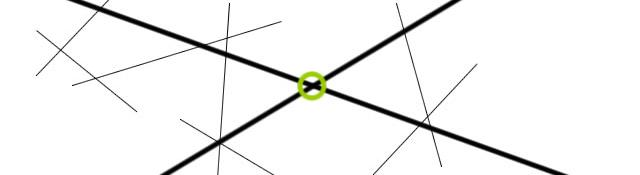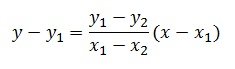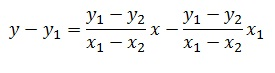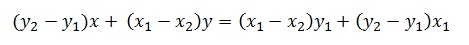## Solving for intersection of lines efficiently

One of the most common tasks done with lines is detecting their intersection. For some reason, not many people know how to do that on a computer. In this post, I assume you have some lines. You got them from some algorithm, or the user supplied them, or whatever. And you want to find the intersection of these lines.

## Step 1: Converting lines to Ax + By = C

Ideally, the lines should be in the form Ax + By = C. But usually, that is not the case. But it is simple enough to convert it into this form. This is done using the two point form of the line.We can generate two points on the line using the given form, And then plug those points into the above equation. And thus you'll get the equation in the form we want.

Rearranging the two-point equation, we get: (just try and understand them... they're not as horrible as they seem :P)

On opening the brackets on the right hand, we get:And multiplying by (x1-x2) and rearranging, we get:Compare this to Ax + By = C... and you'll see that we have our equation!

• A = y2 - y1
• B = x1 - x2
• C = By1 + Ax1

So, convert all your lines into this form, and we're ready for step 2. Actually figuring out intersection points.

## Step 2: Solving for intersection

Right now, we have several lines in the form Ax + By = C. So "solving for intersection" is just solving a set of equations:

A1x + B1y = C1 A2x + B2y = C2

To solve, multiply the first equation with B2 and the second with B1. Then you end up with:

A1B2x + B1B2y = B2C1 A2B1x + B1B2y = B1C2

Subtract the second equation from the first and you get:

A1B2x - A2B1x = B2C1 - B1C2

And thus, we get the x coordinate of intersection:

x = (B2C1 - B1C2) / det

Where det = A1B2 - A2B1

Similarly, you can derive an equation for y. And finally, we arrive at these results:

• x = (B2C1 - B1C2) / det
• y = (A1C2 - A2C1) / det

If det = 0, then the lines are parallel (so you cannot calculate x and y)

## Conclusion

Translate the equations in bullet lists into a program. And you have a program that solves for intersection of lines!

#### Related posts#### 7 unique neural network architectures